• Slides: 21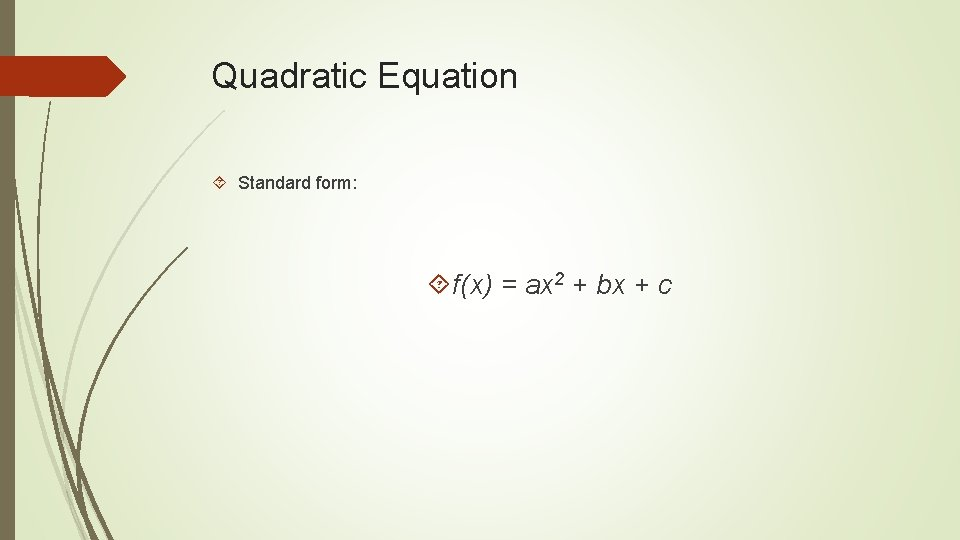Quadratic Equation Standard form: f(x) = ax 2 + bx + cFinite Differences In a linear function, the finite differences are constant. In an exponential function, the finite differences are not constant, but they have a constant common ratio. For a quadratic function, the first finite differences are not constant. They do have a pattern in that they increase or decrease by the same number. The second finite differences are constant.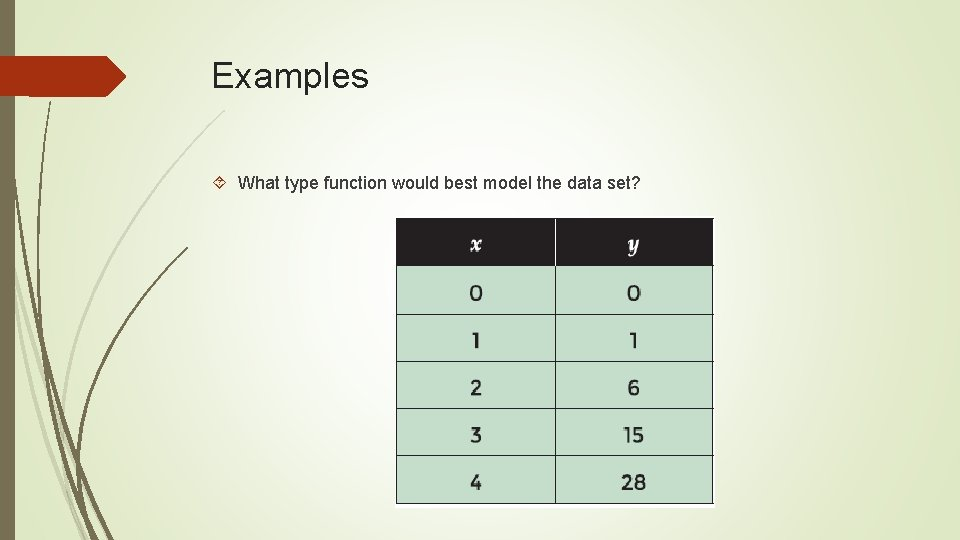Examples What type function would best model the data set?Examples Step 1: Determine whether or not the data represents a linear function Finite differences are not constant; not linear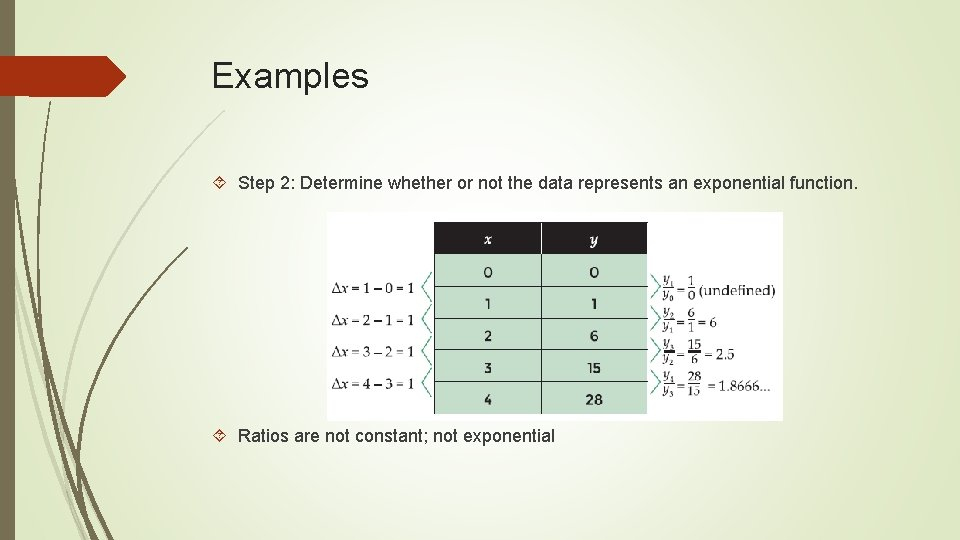Examples Step 2: Determine whether or not the data represents an exponential function. Ratios are not constant; not exponential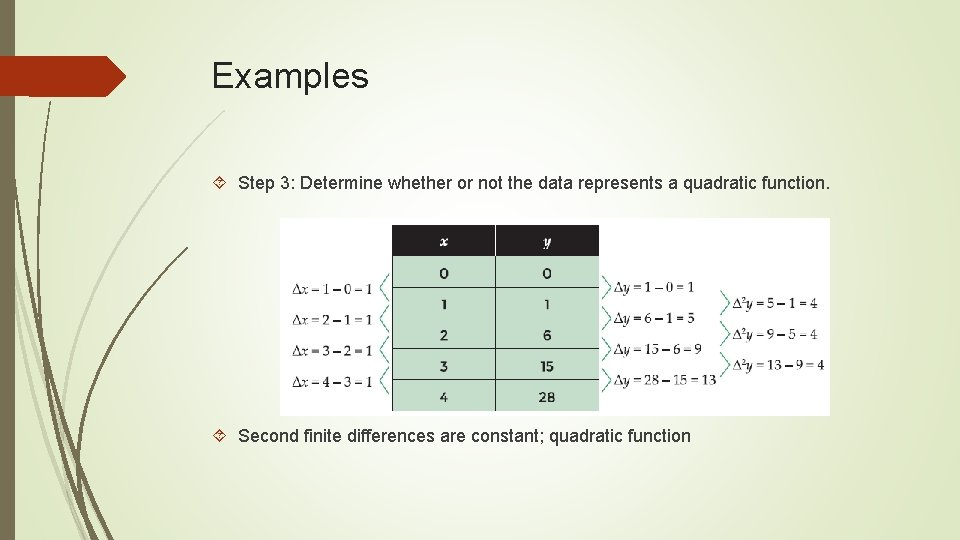Examples Step 3: Determine whether or not the data represents a quadratic function. Second finite differences are constant; quadratic function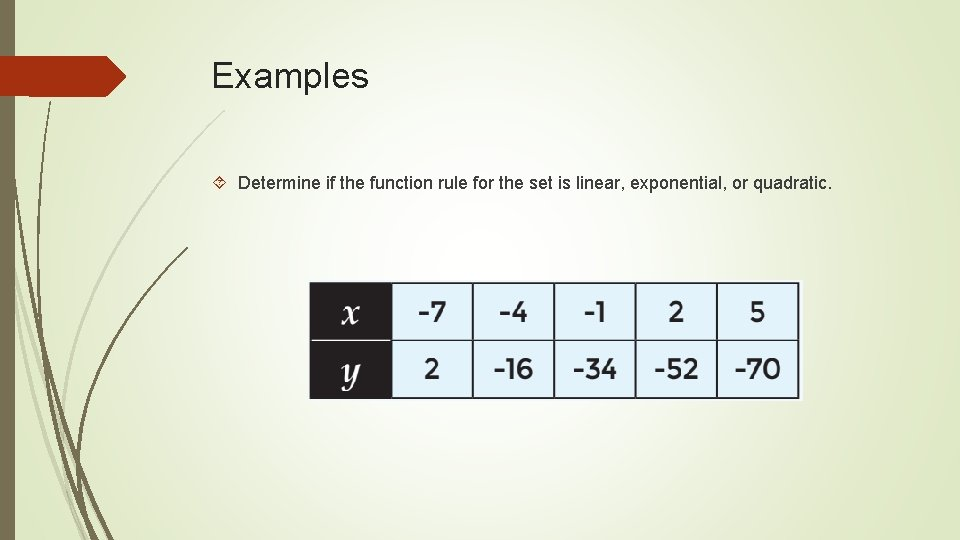Examples Determine if the function rule for the set is linear, exponential, or quadratic.Examples Determine if the function rule for the set is linear, exponential, or quadratic.Writing Quadratic Functions You can use these three patterns to determine the quadratic function from the table of data. The value of c is the y-intercept (y when x = 0) The second difference is equal to 2 a The first difference between the y-values for x = 0 and x = 1 is equal to a + b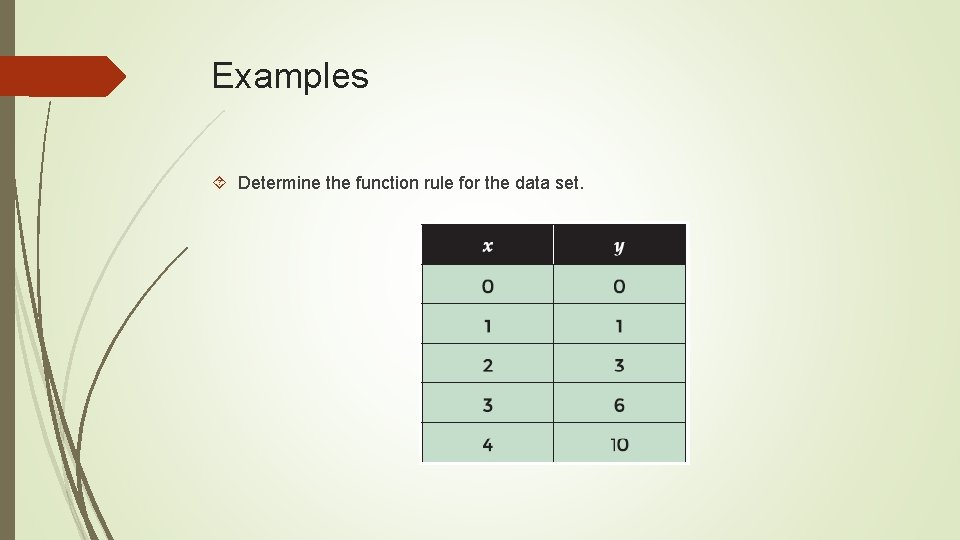Examples Determine the function rule for the data set.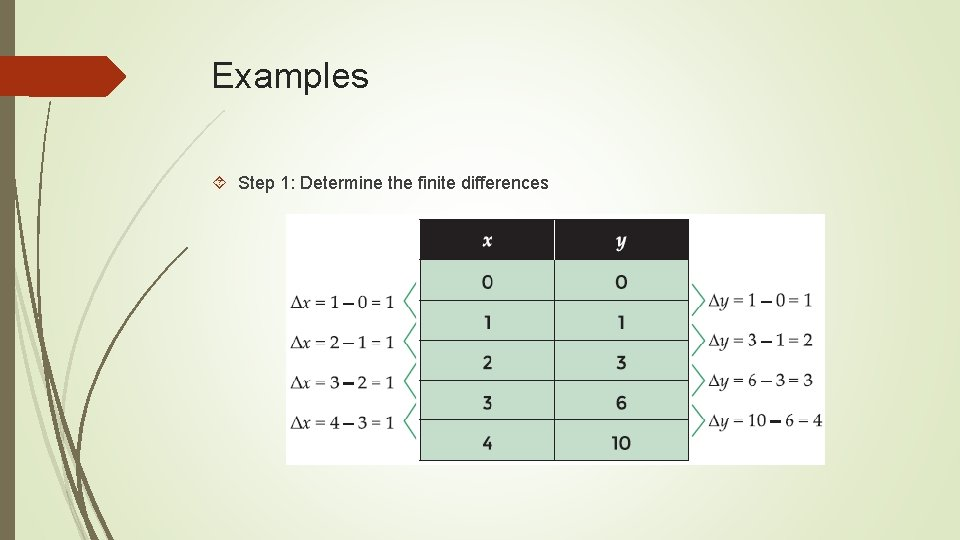Examples Step 1: Determine the finite differences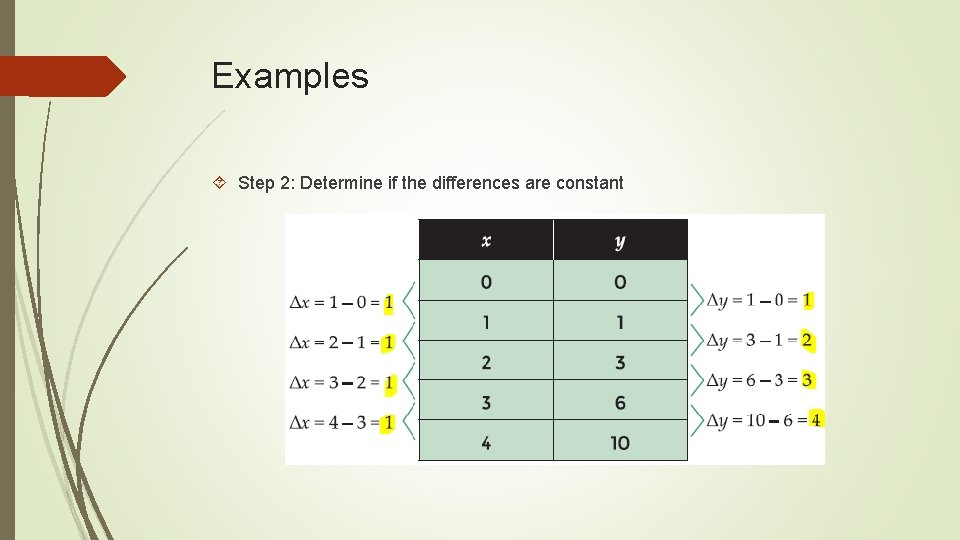Examples Step 2: Determine if the differences are constantExamples Step 3: Determine whether or not the second finite differences are constantExamples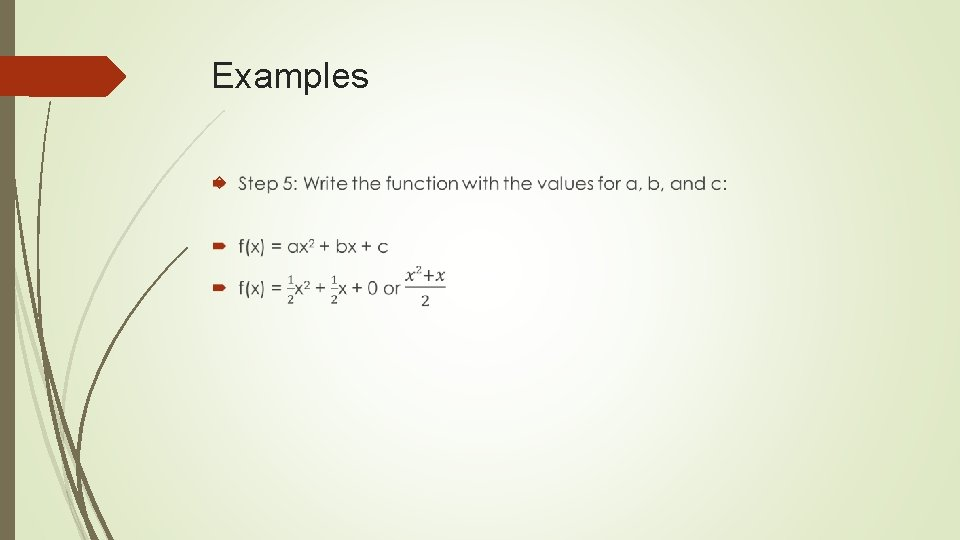Examples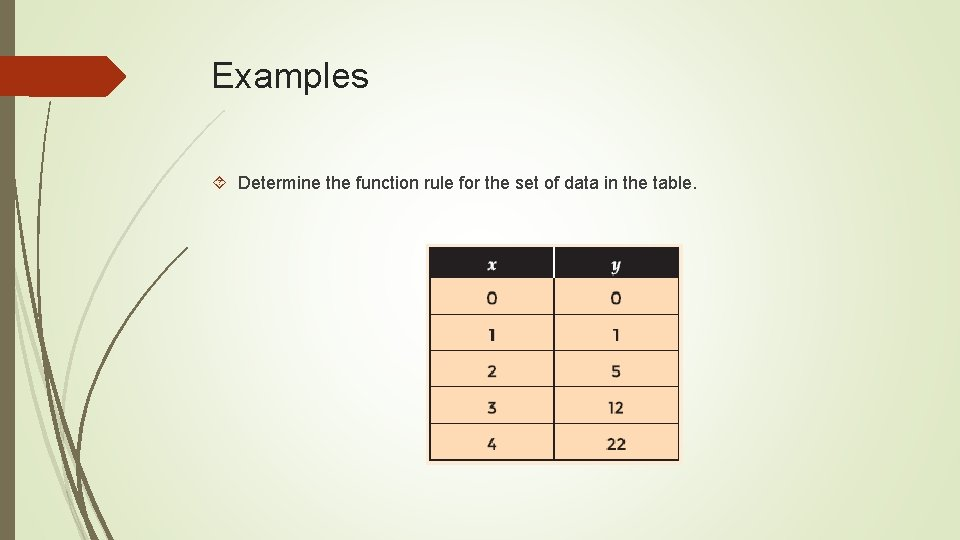Examples Determine the function rule for the set of data in the table.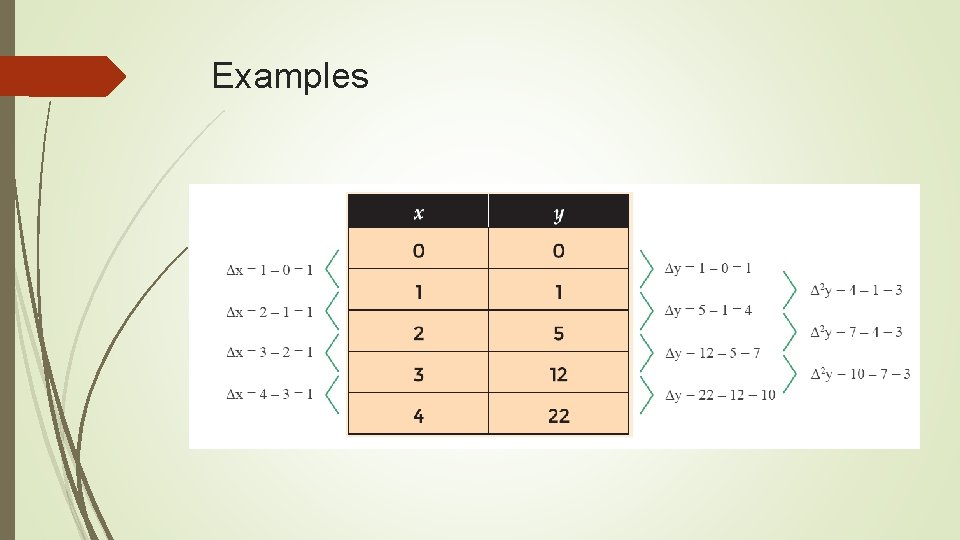ExamplesExamplesExamples Write a quadratic function where the second finite difference is 4, the y-intercept is (0, 1), and a + b = 5Examples Write a quadratic function where the second finite difference is 4, the y-intercept is (0, 1), and a + b = 5 The second finite difference is 4; 2 a = 4, so a = 2 a + b = 5, and a = 2, so b = 3; y-intercept = 1 = c f(x) = 2 x 2 + 3 x + 1